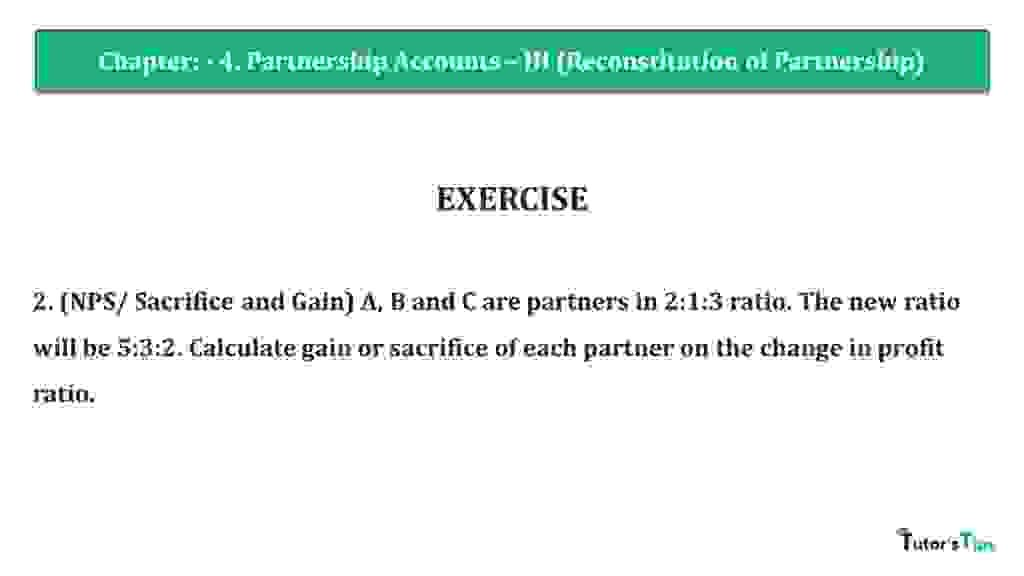# Question 02 Chapter 4 of +2 Part-1 – USHA Publication 12 Class Part – 1Question 02 Chapter 4 of +2-Part-1

2. (NPS/ Sacrifice and Gain) A, B and C are partners in 2:1:3 ratio. The new ratio will be 5:3:2. Calculate the gain or sacrifice of each partner on the change in profit ratio.

### The solution of Question 02 Chapter 4 of +2 Part-1: –

 Old Ratio of A, B & C = 2: 1 : 3 New Ratio of A, B & C = 5 : 3: 2

Calculate the Sacrificing or Gaining Ratio of Partners

Sacrificing or Gaining Ratio = Old Ratio – New Ratio

 A’s Gain = 2 – 5 6 10
 = 10 – 15 30
 = -5 (Gain) 30

 B’s Gain = 1 – 3 6 10
 = 5 – 9 30
 = -4 (Gain) 03

 C’s Sacrifice = 3 – 2 6 10
 = 15 – 6 30
 = 9 (Sacrifice) 03